# Clock

What distance will pass end of 8 cm long hour hand for 15 minutes?

x =  10.5 mm

### Step-by-step explanation: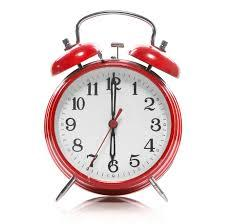Did you find an error or inaccuracy? Feel free to write us. Thank you!Tips to related online calculators
Check out our ratio calculator.
Do you want to convert length units?
Do you want to convert time units like minutes to seconds?

#### You need to know the following knowledge to solve this word math problem:

We encourage you to watch this tutorial video on this math problem:

## Related math problems and questions:

• The small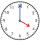The small hand of the wall clock is 15 cm long. What a long journey the end of the hour hand in 15 minutes will describe. (b - in 2 hours)?
• ClocksHow will long path walk the end of the clock's minute-hand long 7 cm for 330 minutes? How long will walk in the same time hour-hand?
• Minute handWhat is the distance the minute hand of the clock travels in 12 minutes, if the diameter of the clock is 30 cm and the hand extends to a distance of 2 cm from the edge of the clock?
• Minute-hand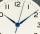How long distance will travel a large (minute) hand on the clock for 48 minutes, if its length is 56 cm?
• Clock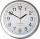How long is the trajectory of the second hand of hours for day, if it is 15 mm long?
• ClocksHow long track travel the second hand of a clock in 46 hours, which is 4 cm long.
• Clocks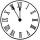What distance will describe the tip of minute hand 6 cm long for 20 minutes when we know the starting position with finally enclose hands each other 120°?
• Little hand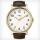What angle shifted little hand on the clock after one hour and 38 minutes?
• Time clockWhat are the angle of the hour hand and the minute hand if it is 0:40?
• ClocksThe length of the minute hand is 12 cm. What track in meters will its end point describe in a week?
• Speed of clockIn how many minutes will the hour hand pass a straight angle and a right angle?
• Clock handsThe second hand has a length of 1.5 cm. How long does the endpoint of this hand travel in one day?
• Special watchFero bought a special watch on the market. They have only one (minute) hand and a display that shows which angle between the hour and minute hand. How many hours was his watch shown - the minute hand points to number 2; the display shows 125°?
• HandsThe clock shows 12 hours. After how many minutes will angle between the hour and minute hand 90°? Consider the continuous movement of both hands hours.
• Look at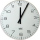Look at the time on the clock now. Information The short hour hand is between 9 and 10. The long minute hand points to 5. Question Second-graders go to recess in 15 minutes. What time will it be then?
• A clockA clock was set right at 6:00 AM. If it gains 3 1/2 minutes per hour, what time will it show at 6:00 PM on the same day? Show your solution
• LunchLunch is given to seniors from 12:15 to 12:40 during the Coronavirus pandemic. What angle will the minute hand of clock describe during this time?